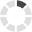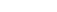### Site InformationLoading... Please wait...

# ECN 221 ECN/221 ECN221 FINAL EXAM (ARIZONA)

\$14.99

## Product Description

ECN 221 ECN/221 ECN221 FINAL EXAM (ARIZONA)

QUESTION 1

1. Concerning the final exam:

 The date and time are listed on the syllabus.  If you fail to show up you will automatically fail the class. The date and time are listed on the syllabus.  If you fail to show up you will still have however many points you earned on homework, other tests and quizzes and will be graded based on those points. The date and time are not on the syllabus. The exam will be administered in the Hayden Library.

1 points

QUESTION 2

1. Concerning your grade in the class:

 Grades will be assigned randomly.  Dr. Cox will use a random number generator to assign points and then make up an entirely new grading scale. Your grade depends on how badly you need a certain score.  If you email Dr. Cox repeatedly asking for a higher grade you will be treated better than other students. You can determine your grade by using the grade calculator that is on Bb and this calculator follows the policy outlined in the syllabus.  All students are treated the same and grades are based on the number of points each student earned by demonstrating their knowledge by answering questions correctly. It is impossible to figure out your grade because the syllabus is unclear and the grading policy is so mysterious.

1 points

QUESTION 3

1. For this assignment you will use data from the World Bank found in the homework data set on the homework 12 tab.  The data on the tab are per capita GDP, per capita health expenditures and the % on young children immunized for DPT and the data are for a few different years and many countries.  Because the World Bank does not have data for every variable for every country for every year there are some missing values in the data.  We will deal with this as we go.  You have opened the tab for the data.

True

False

1 points

QUESTION 4

1. Sort the data by 2013 per capita GDP.  Be careful and make sure that all of the data are kept together.  How many countries have a non-missing entry for per capita GDP?

1 points

QUESTION 5

1. For the countries that have a non-missing per capita GDP what is the average per capita GDP? Answer to the nearest dollar (whole number) and do not include a dollar sign. With all problems you can round up or down.

1 points

QUESTION 6

1. You will run a regression with 2013 per capita GDP and 2013 per capita health care spending.  It seems plausible that in countries where people have greater income the also spend more on health care.  You will use per capita GDP as the independent or explanatory variable in your regression and health care spending will be the dependent variable.  However, excel will error out if there are missing values so use only observations that have both a 2013 per capita GDP and a 2013 per capita spending on health care.  This may mean you either create a new table on a separate tab or delete some of the observations after sorting.  How many observations will you use in your regression?  Hint: it should be 183 and if it isn't then you are going to get wrong numbers later.

1 points

QUESTION 7

1. There is a video on the videos tab in Bb showing how to run a regression in excel.

True

False

0.5 points

QUESTION 8

1. If you are struggling with this homework and/or want to check your knowledge before submitting then you can take advantage of the practice homework.

True

False

0.5 points

QUESTION 9

1. Warning:  I have seen students in the past mix up the dependent and independent variables and this leads to a bunch of wrong answers.  Please be careful and remember that the "Y" variable is the dependent variable and that the "X" variable is the independent variable.  Use the World Bank data provided to run a regression where 2013 per capita health care expenditure is the dependent variable and 2013 per capita GDP is the independent variable.  What is R square?  Answer to three decimal places.

1 points

QUESTION 10

1. Use the results from the regression you just ran with per capita GDP and health care.  You already found R square for this regresion.  What is the estimated variance of the error term?  Answer to the nearest integer.  Hint this number should be less than 1,000,000.

2 points

QUESTION 11

1. Use the results from the regression you just ran with per capita GDP and health care.  You already found R square for this regresion.  What is the estimated standard deviationof the error term?  Answer to the nearest integer.

2 points

QUESTION 12

1. Using the same regression results what is the estimated coefficient on per capita GDP?  Answer to three decimal places.

2 points

QUESTION 13

1. Use the same regression results where you already found R square and other results for this regresion.  What is the standard error for the estimated slope of the regression line?  Answer to four decimal places.

2 points

QUESTION 14

1. Use the regression results you have found.  What is the test statistic for a test that the slope of the regression line is 0?  Answer to two decimal places.

2 points

QUESTION 15

1. In the per capita GDP versus per capita health care expenditure regression that you just ran you fail to reject the hypothesis that the slope is 0 (i.e. that the per capita GDP has no impact on the amount spent on health care).

True

False

2 points

QUESTION 16

1. In the regression that you just ran you reject the hypothesis that the intercept is 0 (as usual assume alpha=.05).

True

False

2 points

QUESTION 17

1. Using the results from the regression you ran, what is the predicted per capita spending on health care for a country with per capita GDP of \$20,000? Answer to the nearest integer, do not include a dollar sign, you may round up or down as usual.  Hint your answer should be between 100 and 10,000 which is not much of a hint but it tells you if you are way out of the ballpark.

2 points

QUESTION 18

1. Based on your regression results and your answer in the previous question using the World Bank data, what happens to per capita expenditure on health care as per capita GDP increases?

 The total expenditure stays constant because as the price increases the amount people buy stays the same. The total expenditure decreases.  As the per capita GDP increase people are healthier and so they spend less on health care on average. The expenditure increases.  As per capita GDP increases people tend to spend more on health care. None of the other answers are correct.

2 points

QUESTION 19

1. Which of the following is an assumption we typically make when performing OLS regression?

 That the variance of the error term is constant across values of the independent variable. That the independent variable has mean 0. That the sum of the squared error terms is equal to 0. The derivative of the MSE with respect to the error term is positive.

1 points

## Customers also viewed

• \$18.99• \$18.99• \$12.99• \$9.99• \$18.99## Related Products

• ECN 220 ECN/220 ECN220 Midterm exam \$35.00• ECN 220 ECN/220 ECN220 Week 4 Quiz \$9.99• ECN 220 ECN/220 ECN220 Week 6 Quiz \$9.99• MATH 221 MATH221 Week 8 Final Exam \$20.00• PH 221 Final Exam (GRANTHAM) \$20.00Click the button below to add the ECN 221 ECN/221 ECN221 FINAL EXAM (ARIZONA) to your wish list.